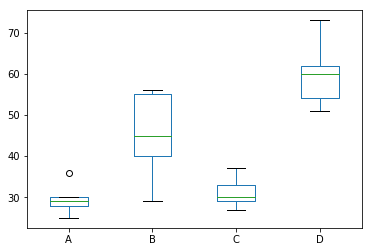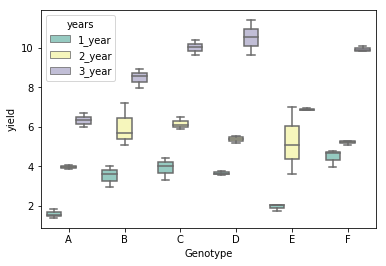# ANOVA using Python

What is ANOVA (ANalysis Of VAriance)?

• used to compare the means of more than 2 groups (t-test can be used to compare 2 groups)
• groups mean differences inferred by analyzing variances
• Main types: One-way (one factor) and two-way (two factors) ANOVA (factor is an independent variable)

Note: In ANOVA, group, factors, and independent variables are similar terms

ANOVA Hypotheses

• Null hypotheses: Groups means are equal (no variation in means of groups)
• Alternative hypotheses: At least, one group mean is different from other groups

ANOVA Assumptions

• Residuals (experimental error) are normally distributed (Shapiro-Wilks Test)
• Homogeneity of variances (variances are equal between treatment groups) (Levene or Bartlett Test)
• Observations are sampled independently from each other

How ANOVA works?

• Check sample sizes: equal number of observation in each group
• Calculate Mean Square for each group (MS) (SS of group/level-1); level-1 is a degree of freedom (df) for a group
• Calculate Mean Square error (MSE) (SS error/df of residuals)
• Calculate F-value (MS of group/MSE)

One-way (one factor) ANOVA with Python

Example data for one-way ANOVA analysis tutorial, dataset

A B C D
25 45 30 54
30 55 29 60
28 29 33 51
36 56 37 62
29 40 27 73

Here, there are four treatments (A, B, C, and D), which are groups for ANOVA analysis. Treatments are independent variable and termed as factor. As there are four types of treatments, treatment factor has four levels.

For this experimental design, there is only factor (treatments) or independent variable to evaluate, and therefore, one-way ANOVA method is suitable for analysis.

Useful reading: Data handling using pandas

``````# I am using Python 3
import pandas as pd
# generate a boxplot to see the data distribution by treatments. Using boxplot, we can easily detect the differences
# between different treatments
d.boxplot(column=['A', 'B', 'C', 'D'], grid=False)
````````````# load packages
import scipy.stats as stats
# stats f_oneway functions takes the groups as input and returns F and P-value
fvalue, pvalue = stats.f_oneway(d['A'], d['B'], d['C'], d['D'])
print(fvalue, pvalue)
# 17.492810457516338 2.639241146210922e-05

# get ANOVA table as R like output
import statsmodels.api as sm
from statsmodels.formula.api import ols
# reshape the d dataframe suitable for statsmodels package
d_melt = pd.melt(d.reset_index(), id_vars=['index'], value_vars=['A', 'B', 'C', 'D'])
# replace column names
d_melt.columns = ['index', 'treatments', 'value']
# Ordinary Least Squares (OLS) model
model = ols('value ~ C(treatments)', data=d_melt).fit()
anova_table = sm.stats.anova_lm(model, typ=2)
anova_table

|                |  df   | sum_sq  | mean_sq  |  F       |  PR(>F)  |
|---------------|--------|---------|----------|----------|----------|
| C(treatments) | 3.0    | 3010.95 | 1003.650 | 17.49281 | 0.000026 |
| Residual      | 16.0   | 918.00  | 57.375   | NaN      | NaN      |

# note: if the data is balanced (equal sample size for each group), Type 1, 2, and 3 sums of squares
# (typ parameter) will produce similar results.
``````

Interpretation: The P-value obtained from ANOVA analysis is significant (P<0.05), and therefore, we conclude that there are significant differences among treatments.

Note: If you have unbalanced (unequal sample size for each group) data, you can perform similar steps as described for one-way ANOVA with balanced design (equal sample size for each group).

From ANOVA analysis, we know that treatment differences are statistically significant, but ANOVA does not tell which treatments are significantly different from each other. To know the pairs of significant different treatments, we will perform multiple pairwise comparison (Post-hoc comparison) analysis using Tukey HSD test.

``````# load packages
from pingouin import pairwise_tukey
# perform multiple pairwise comparison (Tukey HSD)
# for unbalanced (unequal sample size) data, pairwise_tukey uses Tukey-Kramer test
m_comp = pairwise_tukey(data=d_melt, dv='value', between='treatments')
print(m_comp)

group1    group2      mean(group1)    mean(group2)    diff       SE  tail               T    P-tukey    hedges
--  --------  --------  --------------  --------------  ------  -------  ---------  ---------  ---------  --------
0  A         B                   29.6            45     -15.4  4.79062  two-sided  -3.21462   0.010718   -1.83635
1  A         C                   29.6            31.2    -1.6  4.79062  two-sided  -0.333986  0.9        -0.19079
2  A         D                   29.6            60     -30.4  4.79062  two-sided  -6.34574   0.001      -3.625
3  B         C                   45              31.2    13.8  4.79062  two-sided   2.88063   0.0274098   1.64556
4  B         D                   45              60     -15    4.79062  two-sided  -3.13112   0.0136793  -1.78865
5  C         D                   31.2            60     -28.8  4.79062  two-sided  -6.01175   0.001      -3.43421

# note; for clarity, I changed the column names for for factors as group1 and group2 (default it will print as
# A and B
``````

Above results from Tukey HSD suggests that except A-C, all other pairwise comparisons for treatments rejects null hypothesis (P-tukey<0.05) and indicates statistical significant differences.

Test ANOVA assumptions

The Shapiro-Wilk test can be used to check the normal distribution of residuals . Null hypothesis: data is drawn from normal distribution.

``````# load packages
import scipy.stats as stats
w, pvalue = stats.shapiro(model.resid)
print(w, pvalue)
# 0.9685019850730896 0.7229772806167603
``````

As the P-value is non significant, we fail to reject null hypothesis and conclude that data is drawn from normal distribution.

As the data is drawn from normal distribution, use Bartlett’s test to check the Homogeneity of variances. Null hypothesis: samples from populations have equal variances.

``````# load packages
import scipy.stats as stats
w, pvalue = stats.bartlett(d['A'], d['B'], d['C'], d['D'])
print(w, pvalue)
5.687843565012841 0.1278253399753447
``````

As the P-value (0.12) is non significant, we fail to reject null hypothesis and conclude that treatments have equal variances.

Levene test can be used to check the Homogeneity of variances when the data is not drawn from normal distribution.

Two-way (two factor) ANOVA with Python

Example data for two-way ANOVA analysis tutorial, dataset

From dataset, there are two factors (independent variables) viz. genotypes and yield in years. Genotypes and years has five and three levels respectively (see one-way ANOVA to know factors and levels).

For this experimental design, there are two factors to evaluate, and therefore, two-way ANOVA method is suitable for analysis. Here, using two-way ANOVA, we can simultaneously evaluate how type of genotype and years affects the yields of plants. If you apply one-way ANOVA here, you can able to evaluate only one factor at a time.

From two-way ANOVA, we can tests three hypotheses 1) effect of genotype on yield 2) effect of time (years) on yield, and 3) effect of genotype and time (years) interactions on yield

``````# load packages
import pandas as pd
import seaborn as sns
# reshape the d dataframe suitable for statsmodels package
# you do not need to reshape if your data is already in stacked format. Compare d and d_melt tables for detail
# understanding
d_melt = pd.melt(d, id_vars=['Genotype'], value_vars=['1_year', '2_year', '3_year'])
# replace column names
d_melt.columns = ['Genotype', 'years', 'value']
Genotype   years  value
0        A  1_year   1.53
1        A  1_year   1.83
2        A  1_year   1.38
3        B  1_year   3.60
4        B  1_year   2.94

# generate a boxplot to see the data distribution by genotypes and years. Using boxplot, we can easily detect the
# differences between different groups
sns.boxplot(x="Genotype", y="value", hue="years", data=d_melt, palette="Set3")
````````````# load packages
import statsmodels.api as sm
from statsmodels.formula.api import ols
# Ordinary Least Squares (OLS) model
# C(Genotype):C(years) represent interaction term
model = ols('value ~ C(Genotype) + C(years) + C(Genotype):C(years)', data=d_melt).fit()
anova_table = sm.stats.anova_lm(model, typ=2)
anova_table

|                      | sum_sq     | df   |     F      |    PR(>F)    |
|----------------------|------------|------|------------|--------------|
| C(Genotype)          | 58.551733  | 5.0  | 32.748581  | 1.931655e-12 |
| C(years)             | 278.925633 | 2.0  | 390.014868 | 4.006243e-25 |
| C(Genotype):C(years) | 17.122967  | 10.0 | 4.788525   | 2.230094e-04 |
| Residual             | 12.873000  | 36.0 | NaN        | NaN          |
``````

Interpretation: The P-value obtained from ANOVA analysis for genotype, years, and interaction are statistically significant (P<0.05). We conclude that type of genotype significantly affects the yield outcome, time (years) significantly affects the yield outcome, and interaction of both genotype and time (years) significantly affects the yield outcome.

Note: If you have unbalanced (unequal sample size for each group) data, you can perform similar steps as described for two-way ANOVA with the balanced design but set `typ=3`. Type 3 sums of squares (SS) is recommended for an unbalanced design for multifactorial ANOVA.

Now, we know that genotype and time (years) differences are statistically significant, but ANOVA does not tell which genotype and time (years) are significantly different from each other. To know the pairs of significant different genotype and time (years), perform multiple pairwise comparison (Post-hoc comparison) analysis using Tukey HSD test.

Similar to one-way ANOVA, you can use Levene and Shapiro-Wilk test to validate the assumptions for homogeneity of variances and normal distribution of residuals.

• three factor designs can be analyzed in a similar way to two-way ANOVA

References

• Seabold, Skipper, and Josef Perktold. “statsmodels: Econometric and statistical modeling with python.” Proceedings of the 9th Python in Science Conference. 2010.
• Virtanen P, Gommers R, Oliphant TE, Haberland M, Reddy T, Cournapeau D, Burovski E, Peterson P, Weckesser W, Bright J, van der Walt SJ. SciPy 1.0: fundamental algorithms for scientific computing in Python. Nature methods. 2020 Mar;17(3):261-72.
• Mangiafico, S.S. 2015. An R Companion for the Handbook of Biological Statistics, version 1.3.2.
• Vallat, R. (2018). Pingouin: statistics in Python. Journal of Open Source Software, 3(31), 1026, https://doi.org/10.21105/joss.01026

How to cite?

Bedre, R. (2018, October 22). ANOVA using Python. https://reneshbedre.github.io/blog/anova.html

If you have any questions, comments or recommendations, please email me at reneshbe@gmail.com

Last updated: July 07, 2020